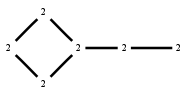# The Markov Bases Database

## G103_bin

The binary graphical model/graph model of G103.

It is a hierarchical model of 6 variables. The dimension of the model is 12.The cardinality of the statespace is 64.

### Properties of the Markov basis

 Markov degree 4 1112

 degree # of gen. 2 4 560 552

The model has the following properties:

• All variables are binary.
• It is a graph model and a graphical model.
• The semigroup is normal.

The following properties of the model are unknown:

• The minimal Markov basis may be unique.### FILES

 Markov basis: sufficient statistics matrix: G103_bin.mar (209.59 kb) G103_bin.mat (3.03 kb) G103_bin.mod (53 b) G103_bin.tar.gz (10.17 kb)

Wrong or missing information? Write us an email.

page last update on March 22 2017. ->Contact